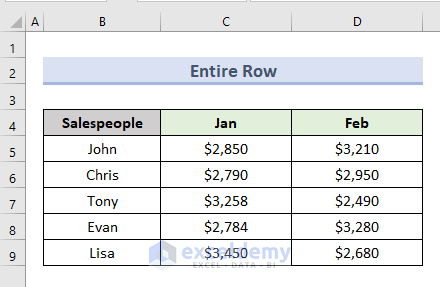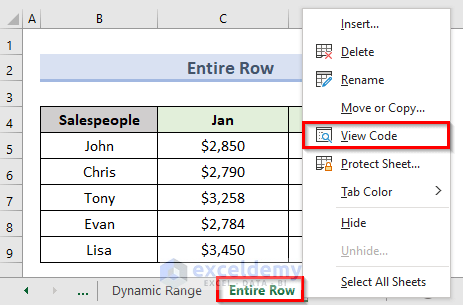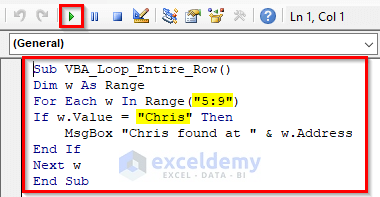# VBA to Loop Through Rows in Range in Excel (6 Examples)

Get FREE Advanced Excel Exercises with Solutions!

In this tutorial, we will cover how to use VBA to loop through rows in range in excel. We utilize loops to prevent doing the same thing over and over again. While working in Microsoft Excel, we may find ourselves in a position where we must perform the same task numerous times. We can do this easily using loops in VBA. In this article, we will demonstrate 6 examples to loop through rows in excel with VBA. The loop that we will use throughout the examples is the ‘For-Next Loop’.

To illustrate the examples of this tutorial, we will use the following dataset. The dataset contains sales amounts for the first two months of 5 Salespeople.

## 1. Using Excel VBA Macro with Range Variable to Loop Through Rows

In the first example, we will use the range variable to loop through rows in range using VBA in excel. We will apply a VBA loop in the following dataset.Let’s see the steps to perform this example.

STEPS:

• To begin with, go to the active worksheet ‘Range Variable’.
• In addition, right-click and select the option ‘View Code’. You can also press Alt + F11 to open it.• The above action opens a code window for that worksheet.
• Furthermore, type the code in the code window:
``````Sub VBA_Loop_through_Rows()
Dim w As Range
For Each w In Range("B5:D9").Rows
w.Cells(1).Interior.ColorIndex = 35
Next
End Sub``````
• Then, click on the Run or press F5 to run the code.• Finally, we will get results like the following screenshot.Read More: Excel VBA: Loop Through Columns in Range

## 2. Applying VBA to Loop Through Rows with Numeric Variable

Another option for looping through rows in a range is to select the variable. In the second example, we will apply VBA in the following dataset to loop through rows in range with numeric variables.Let’s take a look at the steps to do this method.

STEPS:

• First, right-click on the active sheet named ‘Numeric Value’.
• Next, select the option ‘View Code’.• This action will open a code window for that worksheet. You can also press Alt + F11 to open that code window.
• type the following code in that window:
``````Sub VBA_Numeric_Variable()
Dim w As Integer
With Range("B5").CurrentRegion
For w = 1 To .Columns.Count
.Columns(w).NumberFormat = "\$0.00"
Next
End With
End Sub``````
• After that, click on Run or press the F5 key to run the code.• Finally, we can see results like the following picture. The above code changes the format of the number into decimal points.## 3. Applying Macro with User-Selected Range to Loop Through Rows

In the third example, we will use VBA in a user-selected range to loop through rows in a range. So, the user will be able to apply a loop in a selected area of the dataset.Let’s see the steps associated with this example.

STEPS:

• In the beginning, select the cell range (D5:D9).• Next, right-click on the active sheet named ‘User Selected’. Select the option ‘View Code’.• The above command will open a VBA code window for the active worksheet. You can also open that code window by pressing Alt + F11. Insert the following code in that blank code window:
``````Sub VBA_User_Selection()
Dim w As Variant
Set xRange = Selection
For Each w In xRange
MsgBox "Cell value = " & w.Value
Next w
End Sub``````
• Then, to run the code for that worksheet click on the Run or press the F5 key.• So, a message box appears showing the first value of the selected range.• Moreover, if you click on OK it will return the second value of the selected range which is cell D6.• This process will continue until the last value of the selected range which is cell D9.## 4. Performing VBA Loop Through Rows in Dynamic Range

In the fourth example, we will apply VBA to loop through rows in dynamic range. This example is slightly different from the previous ones. We will be able to customize the range in the loop for the excel worksheet. We will use the following dataset to illustrate this method. In the following dataset, we will fill the values of the range (B8:C12) with a specific value.Just go through the below steps to perform this method.

STEPS:

• Firstly, input value 6 in cell B1 and C in cell B2.
• Secondly, right-click on the active cell and select the option ‘View Code’.• This command will open a VBA code window for the active worksheet. Another way to open that code window is by pressing Alt + F11.
• Thirdly insert the following code in that code window:
``````Sub Dynamic_Range()
Dim xRange As String
xRange = "B8:" + Worksheets("Dynamic Range").Cells(2, 2).Value + _
CStr(3 + Worksheets("Dynamic Range").Cells(1, 2).Value)
For Each Row In Range(xRange)
For Each Cell In Row
Cell.Value = "\$2500.00"
Next Cell
Next Row
End Sub``````
• Now, to run the code click on the Run or press the F5 key.• As a result, the dataset fills with the value \$2500.00 in the following way.NOTE:

In the above image, the value 6 represents the first two rows of the range (B8:B9).

• Lastly, input the value 9 in cell B1 instead of 6. We can see the results in the following image.## 5. Inserting Loop Through Entire Row in Excel Range

In the fifth example, we will see how we can apply VBA to loop through the entire row in range. This example will find the position of a specific value from selected one or multiple rows.

###So, let’s see the steps that we will follow to execute this example.

STEPS:

• To begin with, right-click on the active sheet named ‘Entire Row’. Select the option ‘View Code’.

###• The above command opens a blank VBA code window for the active worksheet. We can also get this code window by pressing Alt + F11.
• Next, insert the following code in that code window:
``````Sub VBA_Loop_Entire_Row()
Dim w As Range
For Each w In Range("5:9")
If w.Value = "Chris" Then
MsgBox "Chris found at " & w.Address
End If
Next w
End Sub``````
• Then, click on the Run or press the F5 key to run the code.

###• In the above image, the highlighted value ‘Chris’ indicates the value that we will search for. Range value ‘5:9’ indicates we will search the value in the cell range (B5:B9).
• Finally, a message box displays that the value ‘Chris’ is located in cell B6.

## 6. Using Macro with Loop Through Every n-th Row in Range

In the last example, we will apply VBA to loop through every n-th Row in range. In the following dataset, we will apply color shading to the odd number rows of our data range.So, let’s see the steps to perform this method.

STEPS:

• First, right-click on the active sheet named ‘n-th Row’. Select the option ‘View Code’.• Next, it opens a blank VBA code window for that worksheet. We can also get this code window by pressing Alt + F11.
• Then, type the following code in that code window next:
``````Sub ShadeRows1()
Dim r As Long
With Range("B5").CurrentRegion
For r = 1 To .Rows.Count
If r / 2 = Int(r / 2) Then 'even rows
.Rows(r).Interior.ColorIndex = 43
End If
Next
End With
End Sub``````
• Now, click on the Run or press the F5 key to run the code.• Lastly, we can see that the above code shades only the odd number rows from our dataset.## Conclusion

In conclusion, this tutorial gives you 6 examples of looping through rows in range with VBA in excel. Download the practice worksheet contained in this article to put your skills to the test. If you have any questions, please leave a comment in the box below. Our team will try to respond to your message as soon as possible. Keep an eye out for more inventive Microsoft Excel solutions in the future.

## What is ExcelDemy?

ExcelDemy Learn Excel & Excel Solutions Center provides free Excel tutorials, free support , online Excel training and Excel consultancy services for Excel professionals and businesses. Feel free to contact us with your Excel problems.Mukesh Dipto

Hi there! I am Mukesh Dipto. I graduated with a bachelor's degree in engineering. Currently, I am working as a technical content writer in ExcelDemy. You will find all my articles on Microsoft Excel on this site. Outside of the workplace, my hobbies and interests include sports and meeting new people. I also enjoy sports. My favorite sports are football (to watch and play) and Badminton (play).

We will be happy to hear your thoughtsAdvanced Excel Exercises with Solutions PDF